# U Question 31 1 pts Determine the oxidation state and coordination number of iron in Liz[Fe(CN).l....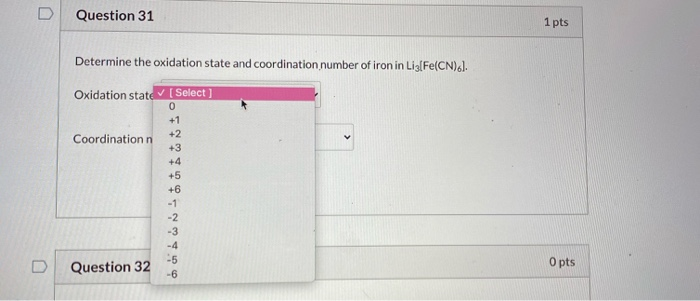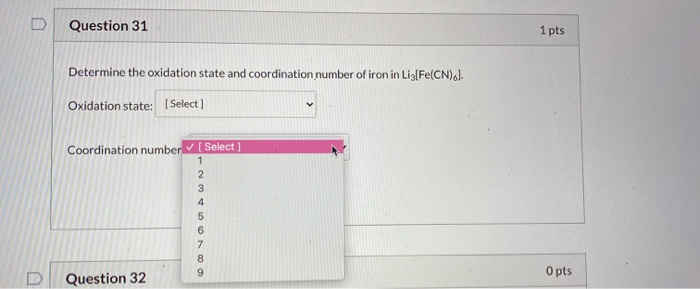U Question 31 1 pts Determine the oxidation state and coordination number of iron in Liz[Fe(CN).l. Oxidation state (Select] 0 +1 +2 Coordination +3 +4 +5 +6 -1 -2 -3 -4 -5 Question 32 -6 O pts
Question 31 1 pts Determine the oxidation state and coordination number of iron in Lis[Fe(CN).). Oxidation state: Select) Coordination number[Select] 1 2 3 4 5 6 7 8 9 Question 32 O pts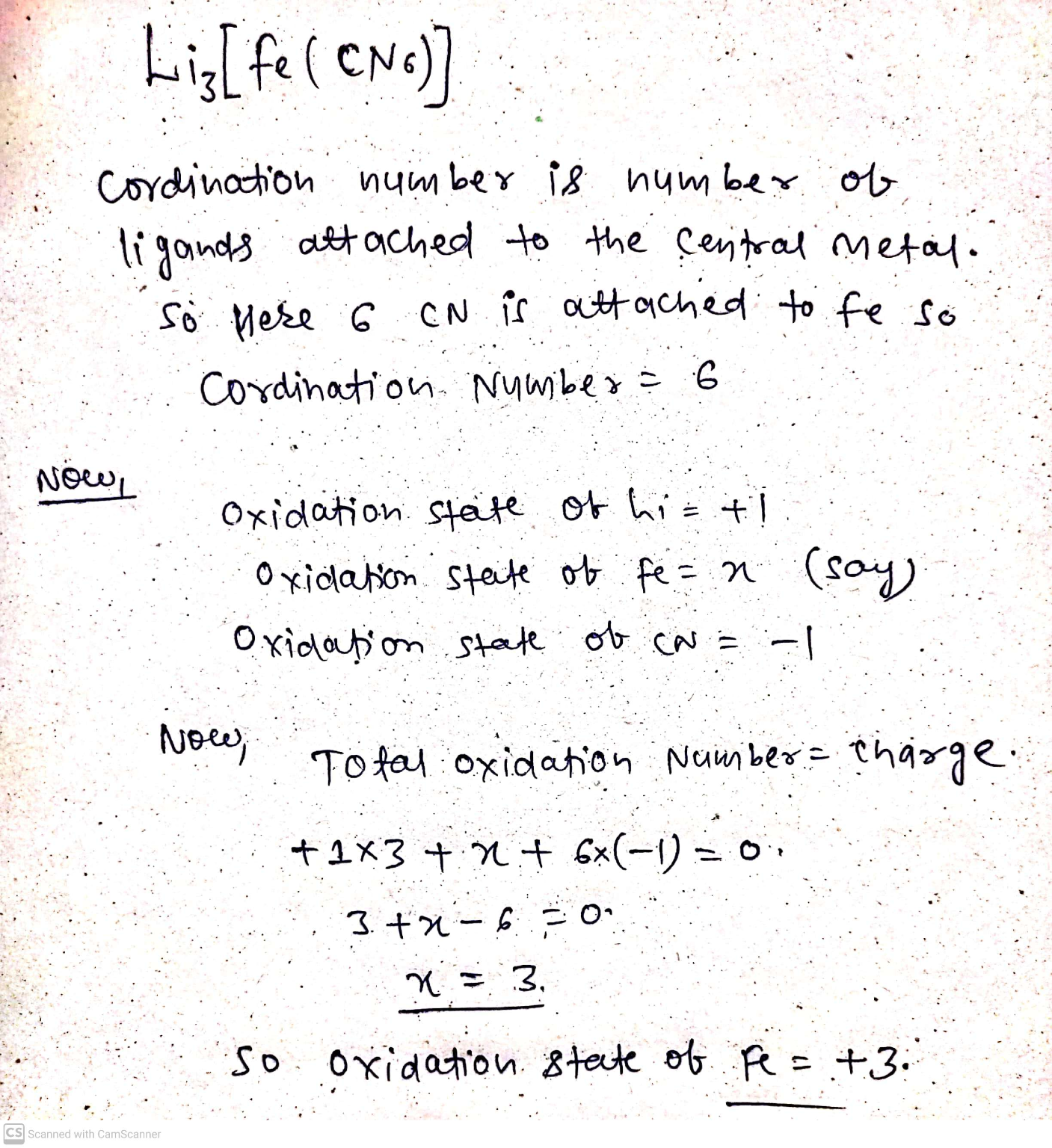hope you understand easily. Please upvote my hardwork and thanks

##### Add Answer of: U Question 31 1 pts Determine the oxidation state and coordination number of iron in Liz[Fe(CN).l....
Similar Homework Help Questions
• ### Question 31 1 pts Determine the oxidation state and coordination number of iron in Lig[Fe(CN)6]. Oxidation...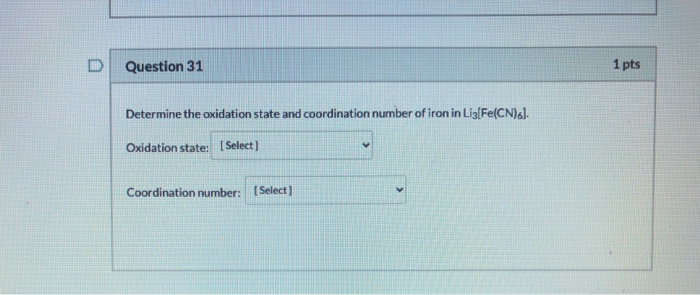Question 31 1 pts Determine the oxidation state and coordination number of iron in Lig[Fe(CN)6]. Oxidation state: (Select) Coordination number: [Select]

• ### Oxidation State and Coordination NUmber

Part A Determine the oxidation state of the metal ion in [Co(NH3)5Br]^2+Part B Determine the coordination number of the metal ion in [Co(NH3)5Br]^2+Part C Determine the oxidation state of the metal ion in [Fe(CN)6]^4-.Part D Determine the coordination number of the metal ion in [Fe(CN)6]^4-.Part E Determine the oxidation state of the metal ion in [Co(ox)3]^4- .Part F Determine the coordination number of the metal ion in [Co(ox)3]^4-

• ### 19 What is the coordination number of the iron in K_3[Fe(CN)_6]? 2 3 4 6 9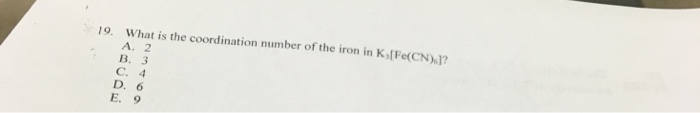19 What is the coordination number of the iron in K_3[Fe(CN)_6]? 2 3 4 6 9

• ### Determine the oxidation and coordination number of the metal ion in each complex ion

Determine the oxidation state and coordination number of the metal ion in each complex ion.a) [Co(NH3)5 Br]2+b) [Fe(CN)6]4-c) [Co(ox)3]4-d) [PdCl4]2-

• ### coordination and oxidation number help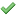Indicate the coordination number of the metal and the oxidationnumber of the metal in each of the following complexes. (Type youranswer for the oxidation number usingthe format +1.)(a) [Mn(CO)5Br]coordination number1oxidation number2(b) [Ni(CN)4]2-coordination number3oxidation number4(c) [Ag(NH3)2]+coordination number5oxidation number6(d) NH4[Cr(NH3)2(NCS)4]coordination number7oxidation number8(e) [Rh(NH3)5Cl](NO3)2coordination number9oxidation number10(f) [Cu(bipy)2I]Icoordination number11oxidation number12

• ### Question 6 7 pts If CN is a strong field ligand, rank the following coordination compounds...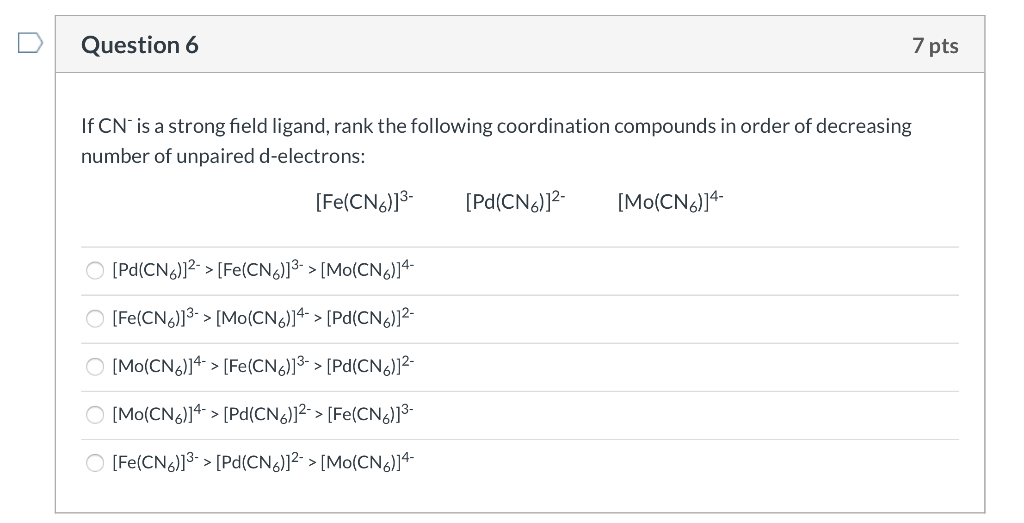Question 6 7 pts If CN is a strong field ligand, rank the following coordination compounds in order of decreasing number of unpaired d-electrons: [Fe(CN6)]3- [Pd(CNG)]2- [Mo(CN)]4- O [Pd(CN)]2-> [Fe(CN6)]3-> [Mo(CN)24- O [Fe(CN)]3-> [Mo(CNG)]4-> [Pd(CN6)]2- O [Mo(CNG)]4-> [Fe(CNG)]3-> [Pd(CNG)]2- [Mo(CN)]4-> [Pd(CN)]2-> [Fe(CN)]3- [Fe(CN6)]3-> [Pd(CN6)]2-> [Mo(CN6)]4-

• ### 2. Indicate i. the metal oxidation state, ii. d-electron count, and iii. coordination number (CN) for...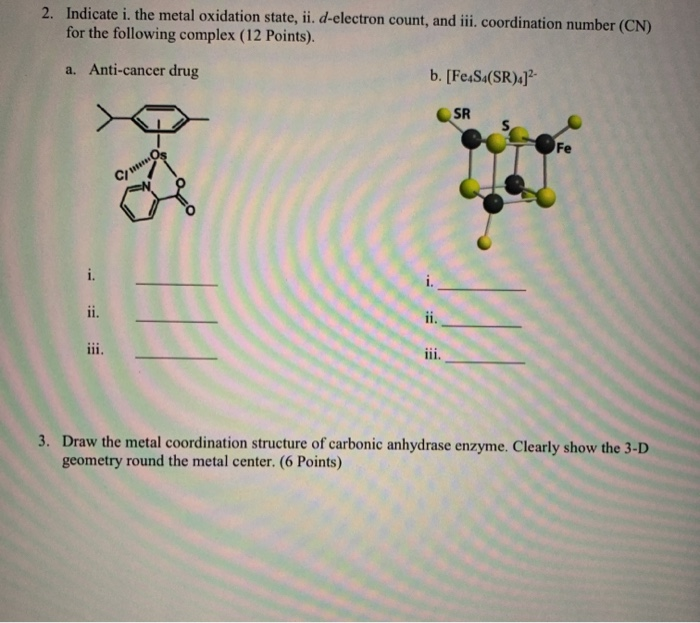2. Indicate i. the metal oxidation state, ii. d-electron count, and iii. coordination number (CN) for the following complex (12 Points). a. Anti-cancer drug b. [Fe S4(SR)] SR Fe COS i. ii. ii. iii. iii. 3. Draw the metal coordination structure of carbonic anhydrase enzyme. Clearly show the 3-D geometry round the metal center. (6 Points)

• ### 2. Indicate i. the metal oxidation state, ii. d-electron count, and iii. coordination number (CN) for...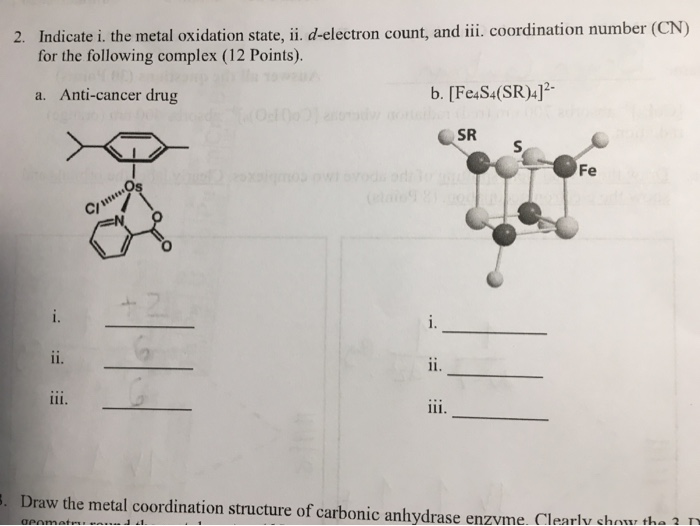2. Indicate i. the metal oxidation state, ii. d-electron count, and iii. coordination number (CN) for the following complex (12 Points). a. Anti-cancer drug b. [Fe4S4(SR)4]2- SR S > Fe Cmos i. i. ii. ii. iii. 5. Draw the metal coordination structure of carbonic anhydrase enzyme. Clearly show the ? geomet 21 Show transcribed image text

• ### Question 7 7 pts If CN is a strong field ligand, rank the following coordination compounds...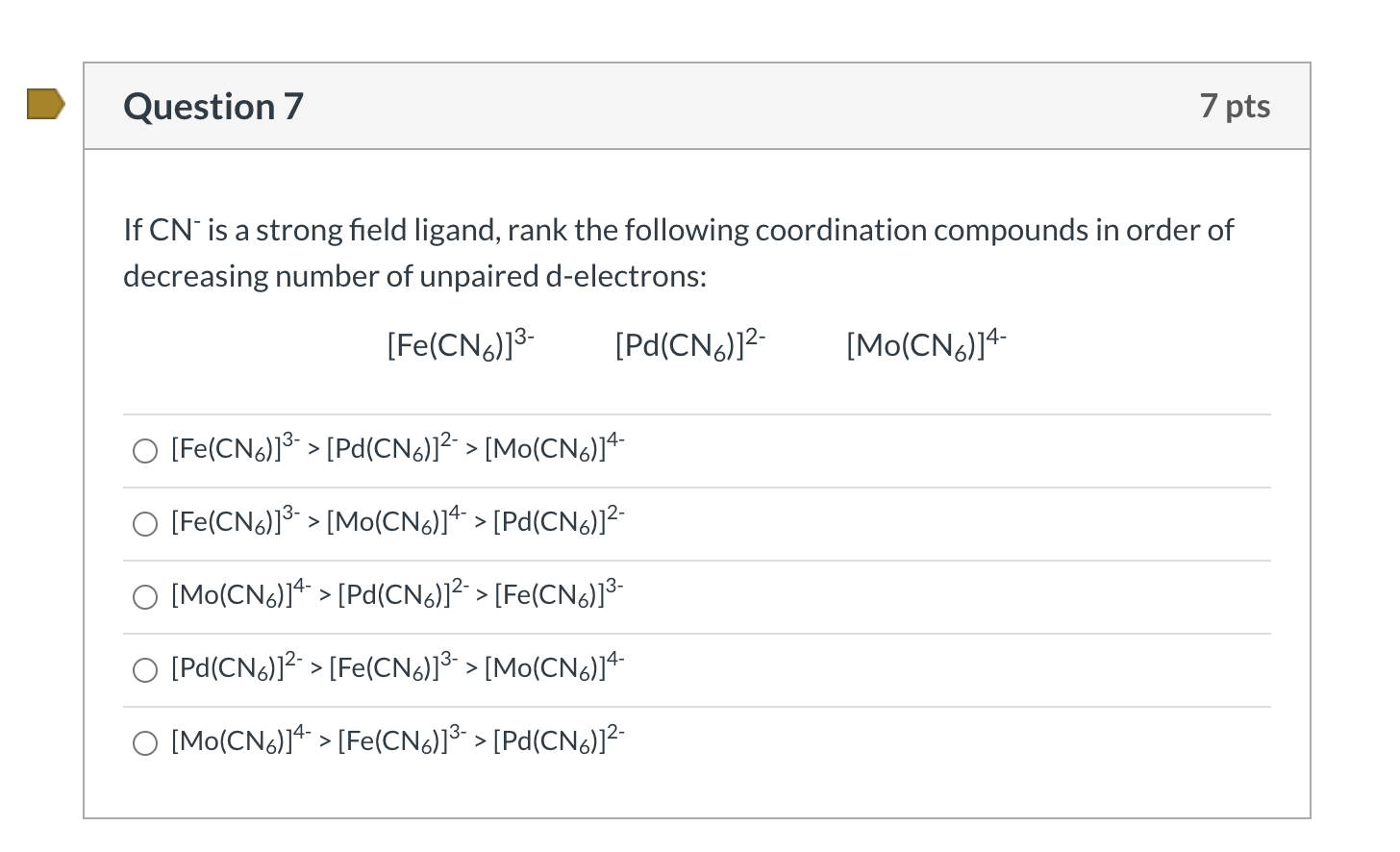Question 7 7 pts If CN is a strong field ligand, rank the following coordination compounds in order of decreasing number of unpaired d-electrons: [Fe(CN6)]3- [Pd(CN6)]2- [Mo(CNG)]4- O [Fe(CN6)]3-> [Pd(CN6)]2-> [Mo(CN6)]4- O [Fe(CN6)]3-> [Mo(CN6)]4- > [Pd(CN6)]2- O [Mo(CN)24-> [Pd(CN)]2-> [Fe(CN)13- O [Pd(CN6)]2-> [Fe(CN6)]3- > [Mo(CN6)]4- O [Mo(CN6)]4- > [Fe(CN6)]3-> [Pd(CN6)]2-

• ### For each of the following transition metal complexes, determine the oxidation state of the metal, its...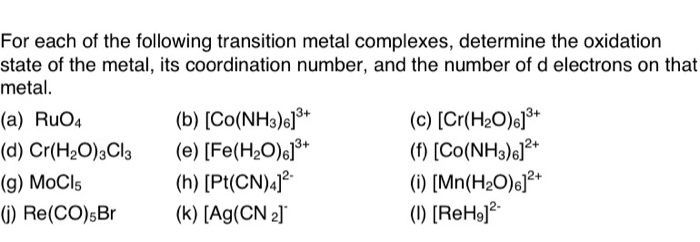For each of the following transition metal complexes, determine the oxidation state of the metal, its coordination number, and the number of d electrons on that metal. (a) RuO4 (b) (CO(NH3).]3+ (c) [Cr(H2O).]8+ (d) Cr(H2O)3Cl2 (e) [Fe(H2O).]3+ (1) (Co(NH3).]2+ (9) MOCI (h) (Pt(CN)4]2- () [Mn(H2O).]2+ (1) Re(CO).Br (k) (Ag(CN 2] (1) [ReH9]?

Free Homework App